# Framing and windowing¶

Contents

## Frames extraction¶

Provides the Frames class to extract frames from raw signals

Extracts overlapping frames from raw (sampled) signals:

array ---> Frames ---> array


Examples

>>> import numpy as np
>>> from shennong.frames import Frames


Build a discrete signal

>>> a = np.arange(10)
>>> a
array([0, 1, 2, 3, 4, 5, 6, 7, 8, 9])


Computes frames of 3s with a shift of 1s (here we assume fs=1Hz for simplicity)

>>> f = Frames(sample_rate=1, frame_shift=1, frame_length=3)
>>> b = f.make_frames(a)
>>> b
array([[0, 1, 2],
[1, 2, 3],
[2, 3, 4],
[3, 4, 5],
[4, 5, 6],
[5, 6, 7],
[6, 7, 8],
[7, 8, 9]])

class shennong.frames.Frames(sample_rate=16000, frame_shift=0.01, frame_length=0.025, snip_edges=True)[source]

Extract frames from raw signals

property sample_rate

Waveform sample frequency in Hertz

Must match the sample rate of the signal specified in process

property frame_shift

Frame shift in seconds

property frame_length

Frame length in seconds

property snip_edges

If true, output only frames that completely fit in the file

When True the number of frames depends on the frame_length. If False, the number of frames depends only on the frame_shift, and we reflect the data at the ends.

property samples_per_frame

The number of samples in one frame

property samples_per_shift

The number of samples between two shifts

get_params(deep=True)

Get parameters for this processor.

Parameters

deep (boolean, optional) – If True, will return the parameters for this processor and contained subobjects that are processors. Default to True.

Returns

params (mapping of string to any) – Parameter names mapped to their values.

property log

Processor logger

abstract property name

Processor name

nframes(nsamples)[source]

Returns the number of frames extracted from nsamples

This function returns the number of frames that we can extract from a wave file with the given number of samples in it (assumed to have the same sampling rate as specified in init).

Parameters

nsamples (int) – The number of samples in the input

Returns

nframes (int) – The number of frames extracted from nsamples

Raises

ValueError – If samples_per_shift == 0, meaning the sample rate is to low w.r.t the frame shift.

set_logger(level, formatter='%(levelname)s - %(name)s - %(message)s')

Change level and/or format of the processor’s logger

Parameters
• level (str) – The minimum log level handled by the logger (any message above this level will be ignored). Must be ‘debug’, ‘info’, ‘warning’ or ‘error’.

• formatter (str, optional) – A string to format the log messages, see https://docs.python.org/3/library/logging.html#formatter-objects. By default display level and message. Use ‘%(asctime)s - %(levelname)s - %(name)s - %(message)s’ to display time, level, name and message.

set_params(**params)

Set the parameters of this processor.

Returns

self

Raises

ValueError – If any given parameter in params is invalid for the processor.

first_sample_of_frame(frame)[source]

Returns the index of the first sample of frame indexed frame

last_sample_of_frame(frame)[source]

Returns the index+1 of the last sample of frame indexed frame

times(nsamples)[source]

Returns an array of (tstart, tstop) times of each frames of a signal

Parameters

nsamples (int) – The number of frames of the considered signal

Returns

times (array, shape = [nframes, 2]) – The start and stop times of each frame extracted from nsamples samples.

boundaries(nframes)[source]

Returns an array of (istart, istop) index boundaries of frames

Parameters

nframes (int) – The number of frames to generate

Returns

boundaries (array, shape = [nframes, 2]) – The start and stop indices of each frame extracted from nsamples samples.

make_frames(array, writeable=False)[source]

Returns an array divided in frames

Parameters
• array (array, shape = [x, ..]) – The array to be divided in frames

• writeable (bool, optional) – Default to False. When True, the returned array is writable but the frames are made of copies of the original array. When False, the result is read-only but this optimizes the process: no explicit copy is made of the orignal array, only views are used. (see https://docs.scipy.org/doc/numpy-1.15.0/reference/generated/ numpy.lib.stride_tricks.as_strided.html)

Returns

frames (array, shape = [nframes(x), samples_per_frame, …]) – The frames computed from the original array

## Windows functions¶

Implementation of different types of window functions

This is usefull when computing frames for features extraction. Uses the kaldi implementation.

The implemented window functions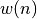are, with length noted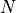:

• rectangular: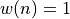• hanning: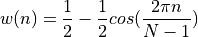• hamming: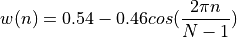• povey (like hamming but goes to zero at edges):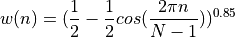• blackman, with blackman_coeff noted as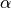: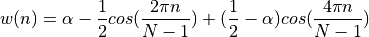Examples

>>> from shennong.window import window
>>> window(5, type='hamming')
array([0.08, 0.54, 1.  , 0.54, 0.08], dtype=float32)
>>> window(5, type='rectangular')
array([1., 1., 1., 1., 1.], dtype=float32)
>>> window(5, type='povey').tolist()
[0.0, 0.5547847151756287, 1.0, 0.5547847151756287, 0.0]

shennong.window.types()[source]

Returns the supported window functions as a list

shennong.window.window(length, type='povey', blackman_coeff=0.42)[source]

Returns a window of the given type and length

Parameters
• length (int) – The size of the window, in number of samples

• type ({'povey', 'hanning', 'hamming', 'rectangular', 'blackman'}) – The type of the window, default is ‘povey’ (like hamming but goes to zero at edges)

• blackman_coeff (float, optional) – The constant coefficient for generalized Blackman window, used only when type is ‘blackman’

Returns

window (array, shape = [length, 1]) – The window with the given type and length

Raises

ValueError – If the type is not valid or length <= 1